#### How Excel handles different data types?

In this article, we will guide you through the basics of Excel, especially the different data types used in Excel.

Excel is designed to handle different data types, including numbers, text, dates, and times. Each data type has its own specific format, and Excel is able to recognize and manipulate these data types based on their formatting.

When you enter data into a cell in Excel, the program automatically determines the data type based on the formatting of the data. For example, if you enter a number with no formatting, Excel will recognize it as a number and allow you to perform mathematical calculations on it. If you enter a date or time, Excel will recognize it as such and allow you to perform calculations and formatting specific to dates and times.

Excel understands four kinds of information:

1. Logical values (True / False or Boolean values)
2. Numeric values (1,23, etc.)
3. Text values (any type of written text)
4. Error Types.

So, when you enter any type of data in a cell and press Enter, Excel recognizes it as one of the data types listed above. For example, you can try to type a number with leading zeroes: 00000100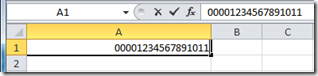After entering it, when either the tab key or enter key is pressed, it just automatically converts it to: 100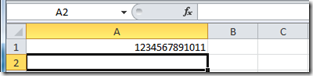Let’s discuss the four types in detail.

## Logical Data type: True or False

Let’s check it with a formula to compare two values: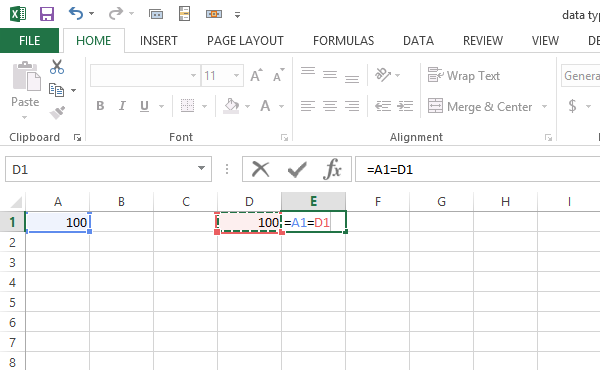The result is as follows: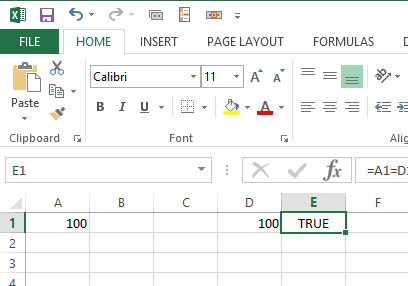So when the condition is analyzed, the result is a Boolean value: True.

## Number Data type

It includes all types of numbers, which can be numeric, floats, or numbers with currency signs, etc. But we can only enter numbers with a maximum of 15 digits.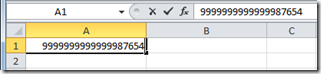The 19 significant digits before Enter turn into 15 significant digits after Enter (truncation happens!)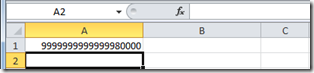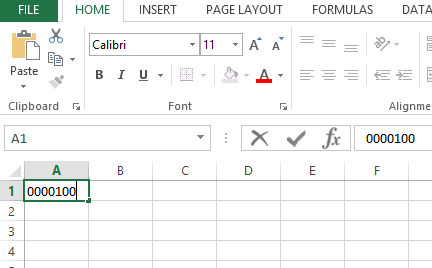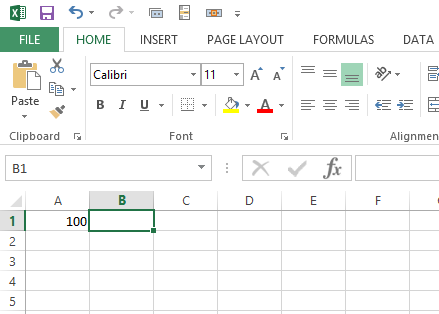`For Excel, 0000100 was a number. If you need these leading zeros, just change the data type to Text.`

## Text Data Type

Excel will recognize a text string of up to 32,768 characters. However, only 1024 can be displayed in a cell.

To check data type just click CTRL + 1.

## Error Data Type

Sometimes when we evaluate a result which has answer in an error e.g dividing with zero (20/0)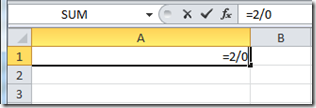The result will be an error type: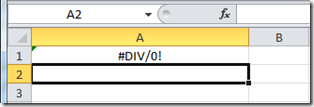Excel is also able to convert between different data types. For example, you can use the VALUE function to convert a text string that represents a number into an actual number that can be used in calculations.

Excel’s ability to handle different data types is a key feature that makes it a powerful tool for data analysis and manipulation. By understanding how Excel handles different data types, you can make the most of its capabilities and perform complex calculations and analyses with ease.